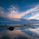1927 lượt xem
1927
A popular strategy which combines Average Directional Index and RSI . In this version, we coded for only LONG entries and exits.
Created by Shanghai Reed Asset Management Co., Ltd.
Bình luận: This strategy is recommended to be used on stocks with daily charts.
```//Copyright by Reed Asset Management registered in Shanghai, China
//该策略为上海蘆田资产管理有限公司制
//@version=2

dilen = input(14, title="DI Length")
dirmov(len) =>
up = change(high)
down = -change(low)
truerange = rma(tr, len)
plus = fixnan(100 * rma(up > down and up > 0 ? up : 0, len) / truerange)
minus = fixnan(100 * rma(down > up and down > 0 ? down : 0, len) / truerange)
[plus, minus]

[plus, minus] = dirmov(dilen)
sum = plus + minus
adx = 100 * rma(abs(plus - minus) / (sum == 0 ? 1 : sum), adxlen)

longEntry1 = sma(close, 20) > sma(close, 20) //check if the ADX is rising
longEntry3 = rsi(close, 14) < 85
longEntry6 = rsi(close, 14) < 50

longCondition1 = longEntry1 and longEntry2 and longEntry3
longCondition2 = longEntry1 and longEntry4 and longEntry5 and longEntry6
if(longCondition1 or longCondition2)
strategy.entry("long", strategy.long)

longExit1 = rsi(close, 9) > 75
longExit5 = sma(close, 20) < sma(close,20)

longExitCondition1 = longExit1 and longExit2 and longExit3
longExitCondition2 = longExit1 and longExit4
longStop1 = strategy.position_avg_price + 4 * tr
longExitCondition3 = longExit5
longStop2 = sma(close, 20)

strategy.close_all(when = longExitCondition1)
if (longExitCondition2)
strategy.exit("exit", "long", stop = longStop1)
if (longExitCondition3)
strategy.exit("exit", "long", stop = longStop2)

//Strategy
```hmm...thanks; I always thought the ADX strategy was when +DI xo -DI to go long.....regardless of whether the ADX line is > 0 and/or it's position relative to 0.2; also interesting that the 20SMA confirms if the ADX is going up from period to period;
Phản hồi